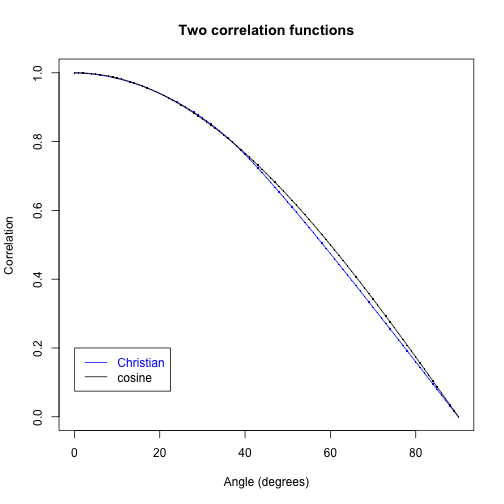### ChaoticUnsharpBall.R

richard — Feb 26, 2014, 8:29 AM

``````## Simulation of the Christian - Roth model
## http://rpubs.com/chenopodium/joychristian

## ... and/or ...

## simulation of Caroline Thompson's chaotic spinning ball, unsharp caps
## http://freespace.virgin.net/ch.thompson1/Papers/The%20Record/TheRecord.htm

## Angular radius of the four circular caps is a drawing from the distribution
## of R = arc cos((sin(Theta)^1.32)/3.16) where Theta ~ Unif(0, pi/2)

## This particular choice is the Christian - Roth innovation.

## This code is copy-paste from http://rpubs.com/chenopodium/joychristian
## My only changes:
## Increase sample size M from 10^6 to 10^7 (sqrt 10 higher precision)
## Only look at angles between 0 and 90 degrees
## (save time, and increase resolution of plot:
## magnification 2x vertically, 4x horizontally).

## Thanks especially to Joy and Chantal, but also to Michel, Daniel and others

set.seed(2938)

M <- 10^7

theta <- runif(M, 0, pi/2)
s <- (sin(theta)^1.32)/3.16
R <- acos(s)*180/pi

hist(R, breaks=1000,xlim=c(70,90), freq=FALSE,
main = "The cap radius distribution",
````````````
angles <- seq(from = 0, to = 90, by = 1) * 2 * pi/360

K <- length(angles)
corrs <- numeric(K)  ## Container for correlations
Ns <- numeric(K)  ## Container for number of states

beta <- 0 * 2 * pi/360  ## Measurement direction 'b' fixed, in equatorial plane

M <- 10^7

z <- runif(M, -1, 1)
t <- runif(M, 0, 2 * pi)
r <- sqrt(1 - z^2)
x <- r * cos(t)

e <- rbind(z, x)  ## 2 x M matrix

b <- c(cos(beta), sin(beta))  ## Measurement vector 'b'

for (i in 1:K) {
alpha <- angles[i]
a <- c(cos(alpha), sin(alpha))  ## Measurement vector 'a'
ca <- colSums(e * a)  ## Inner products of cols of 'e' with 'a'
cb <- colSums(e * b)  ## Inner products of cols of 'e' with 'b'
good <- abs(ca) > s & abs(cb) > s  ## Select the 'states'
N <- sum(good)
corrs[i] <- sum(sign(ca[good]) * sign(cb[good]))/N
Ns[i] <- N
}

plot(angles * 180/pi, corrs, type = "l", col = "blue", main = "Two correlation functions",
xlab = "Angle (degrees)", ylab = "Correlation")
points(angles * 180/pi, corrs, col = "blue", pch = ".", cex = 2)
lines(angles * 180/pi, cos(angles), col = "black")
points(angles * 180/pi, cos(angles), col = "black", pch = ".", cex = 2)

legend(x = 0, y = 0.2, legend = c("Christian", "cosine"), text.col = c("blue",
"black"), lty = 1, col = c("blue", "black"))
``````